# ML Aggarwal Class 7 Solutions for ICSE Maths Chapter 8 Algebraic Expressions Ex 8.1

## ML Aggarwal Class 7 Solutions for ICSE Maths Chapter 8 Algebraic Expressions Ex 8.1

Question 1.
From the algebraic expressions using variables, constants, and arithmetic operations:
(i) 6 more than thrice a number x.
(ii) 5 times x is subtracted from 13.
(iii) The numbers x and y both squared and added.
(iv) Number 7 is added to 3 times the product of p and q.
(v) Three times of x is subtracted from the product of x with itself.
(vi) Sum of the numbers m and n is subtracted from their product.
Solution:Question 2.
A taxi charges ₹ 9 per km and a fixed charge of ₹ 50. If the taxi is hired for x km, write an algebraic expression for this situation.
Solution:Question 3.
Write down the algebraic expression whose terms are:
(i) 5a, -3b, c
(ii) x2, -5x, 6
(iii) x2y, xy, -xy2
Solution:Question 4.
Write all the terms of each of the following algebraic expressions:
(i) 3 – 7x
(ii) 2 – 5a + $$\frac { 1 }{ 2 }$$ b
(iii) 3x5 + 4y3 – 7xy2 + 3
Solution:Question 5.
Identify the terms and their factors in the algebraic expressions given below:
(i) -4x + 5y
(ii) xy + 2x2y2
(iii) 1.2ab – 2.4b + 3.6a
Solution: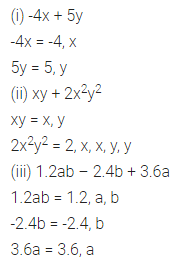Question 6.
Show the terms and their factors by tree diagrams of the following algebraic expressions:
(i) 8x + 3y2
(ii) y – y3
(iii) 5xy2 + 7x2y
(iv) -ab + 2b2 – 3a2
Solution: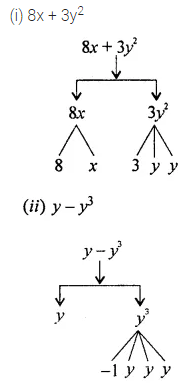Question 7.
Write down the numerical coefficient of each of the following:
(i) -7x
(ii) -2x3y2
(iii) 6abcd2
(iv) $$\frac { 2 }{ 3 }$$ pq2
Solution: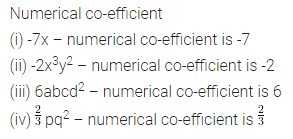Question 8.
Write down the coefficient of x in the following:
(i) -4bx
(ii) 5xyz
(iii) -x
(iv) -3x2y
Solution:Question 9.
In -7xy2z3, write down the coefficient of:
(i) 7x
(ii) -xy2
(iii) xyz
(iv) 7yz2
Solution:Question 10.
Identify the terms (other than constants) and write their numerical coefficients in each of the following algebraic expressions:
(i) 3 – 7x
(ii) 1 + 2x – 3x2
(iii) 1.2a + 0.8b
Solution: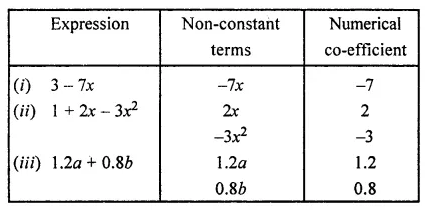Question 11.
Identify the terms which contain x and write the coefficient of x in each of the following expressions:
(i) 13y2 – 8xy
(ii) 7x – xy2
(iii) 5 – 7xyz + 4x2y
Solution: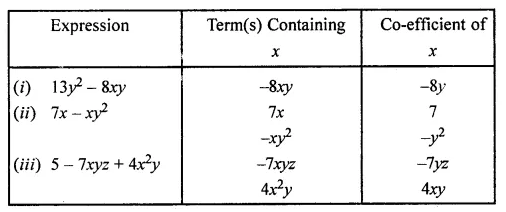Question 12.
Identify the term which contain y2 and write the coefficient of y2 in each of the following expressions:
(i) 8 – xy2
(ii) 5y2 + 7x – 3xy2
(iii) 2x2y – 15xy2 + 7y2
Solution:Question 13.
Classify into monomials, binomials and trinomials:
(i) 4y – 7z
(ii) -5xy2
(iii) x + y – xy
(iv) ab2 – 5b -3a
(v) 4p2q – 5pq2
(vi) 2017
(vii) 1 + x + x2
(viii) 5x2 – 7 + 3x + 4
Solution: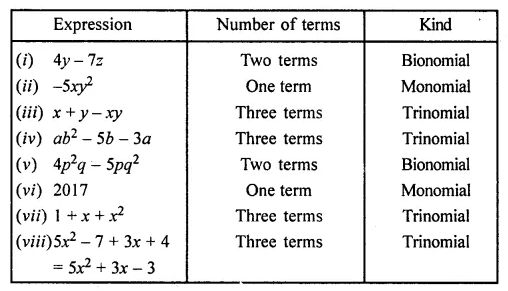Question 14.
State whether the given pair of terms is of like or unlike terms:
(i) -7x, $$\frac { 5 }{ 2 }$$ x
(ii) -29x, -29y
(iii) 2xy, 2xyz
(iv) 4m2p, 4mp2
(v) 12xz, 12x2z2
(vi) -5pq, 7qp
Solution: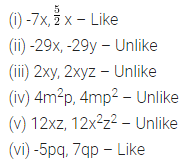Question 15.
Identify like terms in the following:
(i) x2y, 3xy2, -2x2y, 4x2y2
(ii) 3a2b, 2abc, -6a2b, 4abc
(iii) 10pq, 7p, 8q – p2q2, -7qp, -100q, -23, 12q2p2, -5p2, 41, 2405p, 78qp, 13p2q, qp2, 701p2
Solution:Question 16.
Write down the degree of following polynomials in x:
(i) x2 – 6x7 + x8
(ii) 3 – 2x
(iii) -2
(iv) 1 – x2
Solution: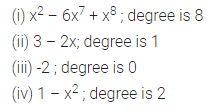Question 17.
Write the degree of the following polynomials:
(i) 3x2 – 5xy2 + 7
(ii) xy2 – y3 + 3y4 – 2
(iii) 7 – 2x3 – 5xy3 + 9y5
Solution:Question 18.
State true or false:
(i) If 5 is constant andy is variable, then 5y and 5 + y are variables
(ii) 7x has two terms, 7 and x
(iii) 5 + xy is a trinomial
(iv) 7a × bc is a binomial
(v) 7x3 + 2x2 + 3x – 5 is a polynomial
(vi) 2x2 – $$\frac { 3 }{ x }$$ is a polynomial
(vii) Coefficient of x in -3xy is -3
Solution: